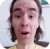FreeKB - Combine merge or join lines in two different variables in BASH
Combine merge or join lines in two different variables in BASHLet's consider a scenario where you need to join or merge lines of data from two different variables. In this example, let's consider two variables that have lines you want to merge.

``````foo="Employee, name=John Doe, occupation=Engineer"
bar="Employee, name=John Doe, department=IT Infrastructure"``````

First, you will need to create unique variable for each field of data in each variable.

``````name=\$(echo \$foo | awk -F',' '{print \$2}')
occupation=\$(echo \$foo | awk -F',' '{print \$3}')
department=\$(echo \$bar | awk -F',' '{print \$3}')``````

Then, you probably will want to create a new variable that contains the merged data.

``result=\$(echo \$name \$occupation \$department)``

In this example, the \$result variable will contain the following data.

``John Doe occupation=Engineer department=IT Infrastructure``

Multiple lines

Additional logic is needed when you are working with variables that contain two or more lines of data.

``````foo="
Employee, name=John Doe, occupation=Engineer
Employee, name=Jane Doe, occupation=Sales Manager
"

bar="
Employee, name=John Doe, department=IT Infrastructure
Employee, name=Jane Doe, department=Sales
"``````

The following script demonstrates the logic.

``````#!/bin/bash

foo="
Employee, name=John Doe, occupation=Engineer
Employee, name=Jane Doe, occupation=Sales Manager
"

bar="
Employee, name=John Doe, department=IT Infrastructure
Employee, name=Jane Doe, department=Sales
"

# Tell bash to split at newline
IFS=\$'\n'

# Create an empty variable that will contain the merged data
merged=""

# Loop through the first variable (\$foo in this example)
for line in \$foo
do

# Create unique variable for each field of data from each line in the \$foo variable
foo_name=\$(echo \$line | awk -F',' '{print \$2}')
occupation=\$(echo \$line | awk -F',' '{print \$3}')

# Loop through the second variable (\$bar in this example)
for data in \$bar
do

# Create unique variable for each field of data from each line in the \$bar variable
bar_name=\$(echo \$data | awk -F',' '{print \$2}')
department=\$(echo \$data | awk -F',' '{print \$3}')

# This if statement is used so that we only process lines in \$foo and \$bar that contain the same name
if [[ \$line =~ \$bar_name ]]; then

# Create a new variable that contains the merged data from \$foo and \$bar
merged+="\$foo_name, \$occupation, \$department"

merged+=\$'\n'
fi
done
done

# Loop through each line
for line in \$merged
do
echo \$line
done

# Cleanup
unset IFS``````

Running this script will produce a variable that contains the merged data.

``````name=John Doe, occupation=Engineer, department=IT Infrastructure
name=Jane Doe, occupation=Sales Manager, department=Sales``````

We will never share your name or email with anyone. Enter your email if you would like to be notified when we respond to your comment.

Please enterin the box below so that we can be sure you are a human.